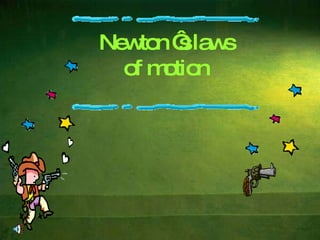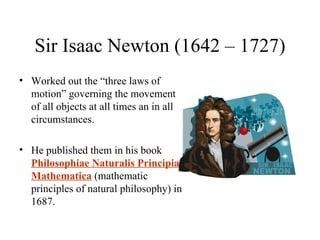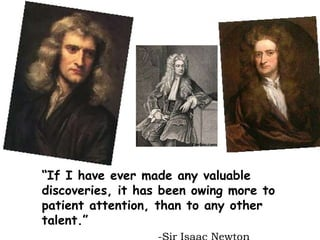Successfully reported this slideshow.

# newton's laws of motion

166

Share×
1 of 30
1 of 30

# newton's laws of motion

166

Share

## More Related Content

### Related Books

Free with a 14 day trial from Scribd

See all

### Related Audiobooks

Free with a 14 day trial from Scribd

See all

### newton's laws of motion

1. 1. Newton ‘s laws of motion
2. 2. Sir Isaac Newton (1642 – 1727) <ul><li>Worked out the “three laws of motion” governing the movement of all objects at all times an in all circumstances. </li></ul><ul><li>He published them in his book Philosophiae Naturalis Principia Mathematica (mathematic principles of natural philosophy) in 1687. </li></ul>
3. 3. “ If I have ever made any valuable discoveries, it has been owing more to patient attention, than to any other talent.” -Sir Isaac Newton
4. 4. Newton’s First Law An object at rest tends to stay at rest and an object in motion tends to stay in motion unless acted upon by an unbalanced force.
5. 5. Newton’s 1 st Law of Motion
6. 6. What does this mean? <ul><li>Basically, an object will “keep doing what it was doing” unless acted on by an unbalanced force. </li></ul><ul><li>If the object was sitting still, it will remain stationary . If it was moving at a constant velocity, it will keep moving . </li></ul><ul><li>It takes force to change the motion of an object. </li></ul>
7. 7. What is meant by unbalanced force? If the forces on an object are equal and opposite, they are said to be balanced, and the object experiences no change in motion. If they are not equal and opposite, then the forces are unbalanced and the motion of the object changes.
8. 8. Some Examples from Real Life <ul><li>Two teams are playing tug of war. They are both exerting equal force on the rope in opposite directions. This balanced force results in no change of motion. </li></ul>A soccer ball is sitting at rest. It takes an unbalanced force of a kick to change its motion.
9. 9. 1 st Law <ul><li>THE COIN WOULD BE IN REST UNLESS ACTED UPON BY AN UNBALANCED FORCE </li></ul>
10. 10. 1 st Law <ul><li>Once airborne, unless acted on by an unbalanced force (gravity and air – fluid friction), it would never stop! </li></ul>
11. 11. Why was it so difficult to stop the TITANIC from colliding with the iceberg?
12. 12. The mass of the Titanic is very large. Inertia is proportionate to mass. The Titanic could not change its direction because its extremely high inertia forces it to continue in a straight line, thereby colliding with the iceberg. menu
13. 13. Newton’s Second Law The acceleration of an object is directly proportional to the net force acting on it and inversely proportional to its mass.
14. 14. What does F = ma mean? <ul><li>Force is directly proportional to mass and acceleration. Imagine a ball of a certain mass moving at a certain acceleration. This ball has a certain force. </li></ul>Now imagine we make the ball twice as big (double the mass) but keep the acceleration constant. F = ma says that this new ball has twice the force of the old ball. Now imagine the original ball moving at twice the original acceleration. F = ma says that the ball will again have twice the force of the ball at the original acceleration.
15. 15. More about F = ma <ul><li>If you double the mass, you double the force. If you double the acceleration, you double the force. </li></ul><ul><li>What if you double the mass and the acceleration? </li></ul><ul><li>(2m)(2a) = 4F </li></ul><ul><li>Doubling the mass and the acceleration quadruples the force. </li></ul><ul><li>So . . . what if you decrease the mass by half ? How much force would the object have now? </li></ul>
16. 16. What does F = ma say? F = ma basically means that the force of an object comes from its mass and its acceleration. Something very small (low mass) that’s changing speed very quickly (high acceleration), like a bullet, can still have a great force. Something very small changing speed very slowly will have a very weak force. Something very massive (high mass) that’s changing speed very slowly (low acceleration), like a glacier, can still have great force.
17. 17. What is the function of the hand glove of this baseball player?
18. 18. The hand glove increases the time of the collision, thereby reducing the force. F = ma = m (v-u) t menu Force is inversely proportional to time
19. 19. Check Your Understanding <ul><li>1. What acceleration will result when a 12 N net force applied to a 3 kg object? A 6 kg object? </li></ul><ul><li>  </li></ul><ul><li>2. A net force of 16 N causes a mass to accelerate at a rate of 5 m/s 2 . Determine the mass. </li></ul><ul><li>3. How much force is needed to accelerate a 66 kg skier 1 m/sec/sec? </li></ul>
20. 20. Check Your Understanding <ul><li>1. What acceleration will result when a 12 N net force applied to a 3 kg object? </li></ul><ul><li> 12 N = 3 kg x 4 m/s/s </li></ul><ul><li>  </li></ul><ul><li>2. A net force of 16 N causes a mass to accelerate at a rate of 5 m/s 2 . Determine the mass. </li></ul><ul><li>16 N = 3.2 kg x 5 m/s/s </li></ul><ul><li>  </li></ul><ul><li>3. How much force is needed to accelerate a 66 kg skier 1 m/sec/sec? </li></ul><ul><li>66 kg-m/sec/sec or 66 N </li></ul>
21. 21. Newton’s Third Law <ul><li>For every action there is an equal and opposite reaction. </li></ul>
22. 22. 3 rd Law <ul><li>According to Newton, whenever objects A and B interact with each other, they exert forces upon each other. When you sit in your chair, your body exerts a downward force on the chair and the chair exerts an upward force on your body. </li></ul>
23. 23. 3 rd Law <ul><li>There are two forces resulting from this interaction - a force on the chair and a force on your body. These two forces are called action and reaction forces. </li></ul>
24. 24. 3 rd Law Flying gracefully through the air, birds depend on Newton’s third law of motion. As the birds push down on the air with their wings, the air pushes their wings up and gives them lift. Reaction Force
25. 25. 3 rd Law The reaction of a rocket is an application of the third law of motion. Various fuels are burned in the engine, producing hot gases. The hot gases push against the inside tube of the rocket and escape out the bottom of the tube. As the gases move downward, the rocket moves in the opposite direction.
26. 26. How does a rocket work?
27. 27. Rocket Pushes Gas
28. 28. How does a jet plane work?
29. 29. Review <ul><li>Newton’s First Law: </li></ul><ul><li>Objects in motion tend to stay in motion and objects at rest tend to stay at rest unless acted upon by an unbalanced force. </li></ul><ul><li>Newton’s Second Law: </li></ul><ul><li>Force equals mass times acceleration (F = ma). </li></ul><ul><li>Newton’s Third Law: </li></ul><ul><li>For every action there is an equal and opposite reaction. </li></ul>EXIT
30. 30. T H A N K Y O U By PAVAN KUMAR 09P1254 1 PCME ‘I’